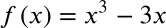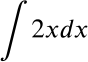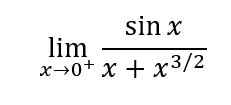Quiz at the end of Chapter 4
1. Over the course of a 4 hour drive, a car traveled 200 miles. Which of the following statements below is a what the "mean value theorem" tells us about this situation?
2. What is a "critical point" of a function?
3. At a hospital, a nurse measures a patient's temperature and determines that it is 98.6°. Twelve hours later, the nurse on the next shift measures that patient's temperature, and she also gets a temperature of 98.6°. According to Rolle's Theorem, the function that describes the patient's temperature for the last 12 hours _______.
4. Suppose we know that the function f(x) has a critical point at x=a. We can determine the derivative of f(x), and we know that f'(x)<0 for x<a and f'(x)>0 for x>a. This is enough information to tell us that _____.
5. When the second derivative of a function is positive at x=a, the function "concave _____" at x=a.
6. The function shown below has two "critical points". Find them:7. Determine the antiderivative of the following function:8. Which of the following is the right way to express an antiderivative as an indefinite integral? Here you should assume that F(x) is the antiderivative of f(x).
9. Evaluate the following indefinite integral:10. Using l'Hopital's Rule, we know that the limit shown below is equal to which of the four expressions:This form was created inside of Evansville Vanderburgh School Corporation. Report Abuse